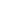We love what we do hereServices01.

UX / UI

• Mobile Design
• Web Design
• Product Design
• UX/UI Consulting02.

Development

• Website
• Mobile Application
• Custom / Enterprise Application
• On Premise & Cloud Based Application
• Software Testing & Maintenance
Our Work
Our work
See all projectsLogic. The Simple Solution.Logic. The Simple Solution.Logic. The Simple Solution.Logic. The Simple Solution.Logic. The Simple Solution.Logic. The Simple Solution.Logic. The Simple Solution.Logic. The Simple Solution.Logic. The Simple Solution.Logic. The Simple Solution.Logic. The Simple Solution.Logic. The Simple Solution.Logic. The Simple Solution.Logic. The Simple Solution.Logic. The Simple Solution.Logic. The Simple Solution.Logic. The Simple Solution.Logic. The Simple Solution.Logic. The Simple Solution.Logic. The Simple Solution.Logic. The Simple Solution.Logic. The Simple Solution.Logic. The Simple Solution.Logic. The Simple Solution.Logic. The Simple Solution.Logic. The Simple Solution.Logic. The Simple Solution.
Logic. The Simple Solution.Logic. The Simple Solution.Logic. The Simple Solution.Logic. The Simple Solution.Logic. The Simple Solution.Logic. The Simple Solution.Logic. The Simple Solution.Logic. The Simple Solution.Logic. The Simple Solution.Logic. The Simple Solution.Logic. The Simple Solution.Logic. The Simple Solution.Logic. The Simple Solution.Logic. The Simple Solution.Logic. The Simple Solution.Logic. The Simple Solution.Logic. The Simple Solution.Logic. The Simple Solution.Logic. The Simple Solution.Logic. The Simple Solution.Logic. The Simple Solution.Logic. The Simple Solution.Logic. The Simple Solution.Logic. The Simple Solution.Logic. The Simple Solution.Logic. The Simple Solution.Logic. The Simple Solution.
Custom Software.UX / UI.Web Development.Mobile App Development.Custom Software.UX / UI.Web Development.Mobile App Development.Custom Software.UX / UI.Web Development.Mobile App Development.Custom Software.UX / UI.Web Development.Mobile App Development.Custom Software.UX / UI.Web Development.Mobile App Development.
Founders
About usFounders